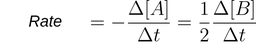The reaction rate is the change in concentration of a reactant or product per unit of time.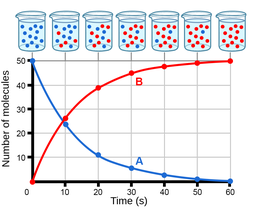Figure 1. The plot of the number of reactant (A) and product (B) molecules over time. The reactant is decreasing while the product is increasing with time.

Take the following reaction as an example: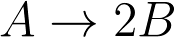The reaction rate can be determined by measuring the rate of disappearance of reactant A.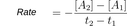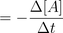• [A2] : Concentration of A at t2 (M)
• [A1] : Concentration of A at t1 (M)
• t2 : Time 2 (s)
• t1 : Time 1 (s)

The units for reaction rate are (mol/L)/s or M/s.

The reaction rate can also be expressed as the rate of appearance of product B.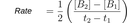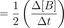• [B2] : concentration of B at t2 (M)
• [B1] : concentration of B at t1 (M)
• t2 : Time 2 (s)
• t1 : Time 1 (s)

With the units of the reaction rate still being (mol/L)/s or M/s.

### Things to consider

Be mindful of the difference between using the reactant (A) vs. product (B) for determining the reaction rate. The value of the reaction rate should be positive, and since [A] decreases with time, Δ[A] is negative. Therefore, we add a negative sign to the formula when expressing the reaction rate as the disappearance of the reactant.

We also need to consider the stoichiometry relationship between product and reactant. In our example, 2 mol of B appears for 1 mol of A. Therefore, the rate of appearance of B is twice the rate of disappearance of A.

The reaction rate formula for our example can be summarized as follows: